MEDIUM
DATA STRUCTURES AND ALGORITHMS

# How to Solve Count Complete Tree Nodes

Written ByHassan JamousTom Wagner

## Count Complete Tree Nodes Introduction

The Count Complete Tree Nodes problem asks us to count the number of nodes in a complete binary tree. This problem requires careful consideration of what a complete binary tree is - where every level besides the last is filled in - and the traversal algorithms necessary to explore the tree efficiently.

## Count Complete Tree Nodes Problem

Given the root of a complete binary tree, return the number of nodes in the tree.

For this problem a binary tree is considered “complete” if every level besides the last level is completely filled in.

### Example Inputs and Outputs

#### Example 1

Input:

``````root = [1, 2]
``````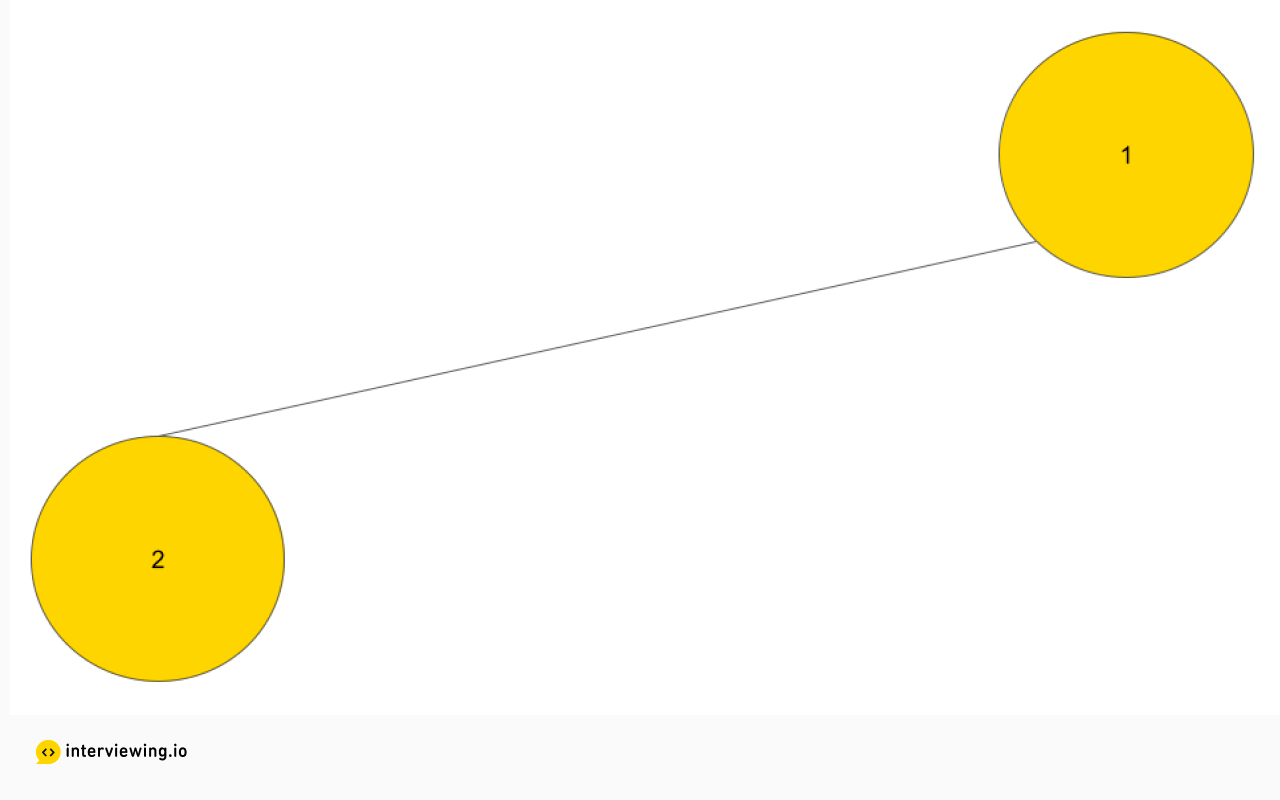Output: 2

#### Example 2

Input:

``````root = [1, 2, 3, 4, 5, 6]
``````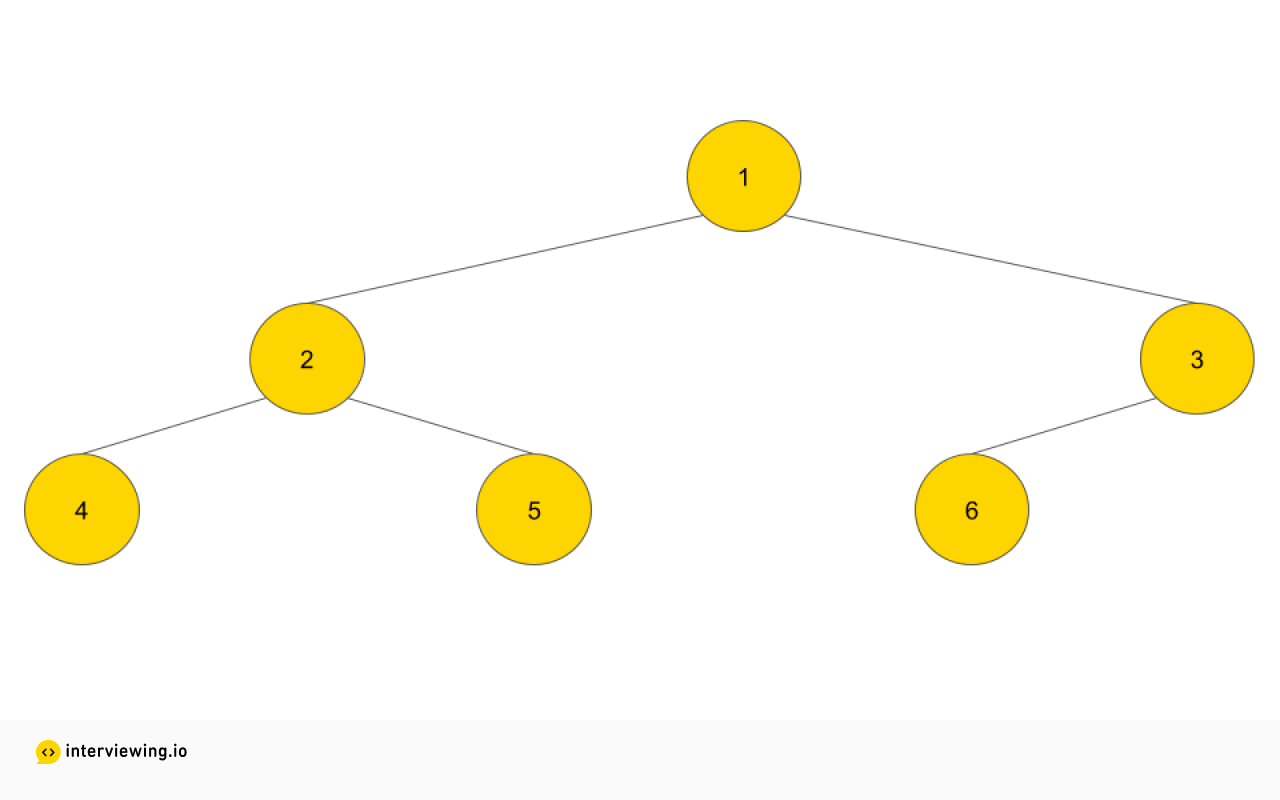Output: 6

Constraints

• The number of nodes is less than 10^5.
• The input is guaranteed to be a complete binary tree.
• Tree size fits in memory.

## Count Complete Tree Nodes Solutions

#### Approach 1: Brute Force

When solving an algorithm, it is often best to start with the brute force solution, and from there you can optimize the solution to make it more efficient.

The brute force solution can be achieved by traversing the whole tree and counting the nodes. There are two main methods to traverse a tree, depth-first search (DFS) or breadth-first search (BFS), and each of those methods has pros/cons depending on the problem at hand. However, when the problem simply requires traversing the tree as a whole with no possibility of exiting the traversal early then either approach will work and you can proceed with whatever implementation you are more comfortable coding.

For this problem, let’s use DFS. DFS starts at the root of the tree and explores as deep as possible along each branch before backtracking. DFS can be implemented iteratively or recursively, and for most people both coding out and reading the recursive implementation is easier, so let’s go with the recursive implementation for the purposes of this problem.

``````# TreeNode definition
# class TreeNode:
#    def __init__(self, data):
#        self.data = data
#        self.left = None
#        self.right = None

def count_nodes_dfs(root: TreeNode):
if root is None:
return 0
return count_nodes_dfs(root.left) + count_nodes_dfs(root.right) + 1
``````

#### Time/Space Complexity

• Time complexity: as we are visiting the whole tree, the time complexity will be `O(n)` where n is the number of nodes.
• Space complexity: `O(h)` where h is the height of the tree. It is not `O(1)` as the recursive function is using the call stack. As this is a complete binary tree, the height would be `FLOOR(log2n)` so space complexity is `O(log2n)`.

We are making progress. We know the brute force solution, we will communicate it with the interviewer, and we will work on improvements.

#### Every Level, Except Possibly The Last, Is Completely Filled

We know that in a complete binary tree, every level, except possibly the last, is completely filled. Let’s take the tree below where we have all levels completely filled. Can we think of a way to find the count of all nodes without traversing the whole tree?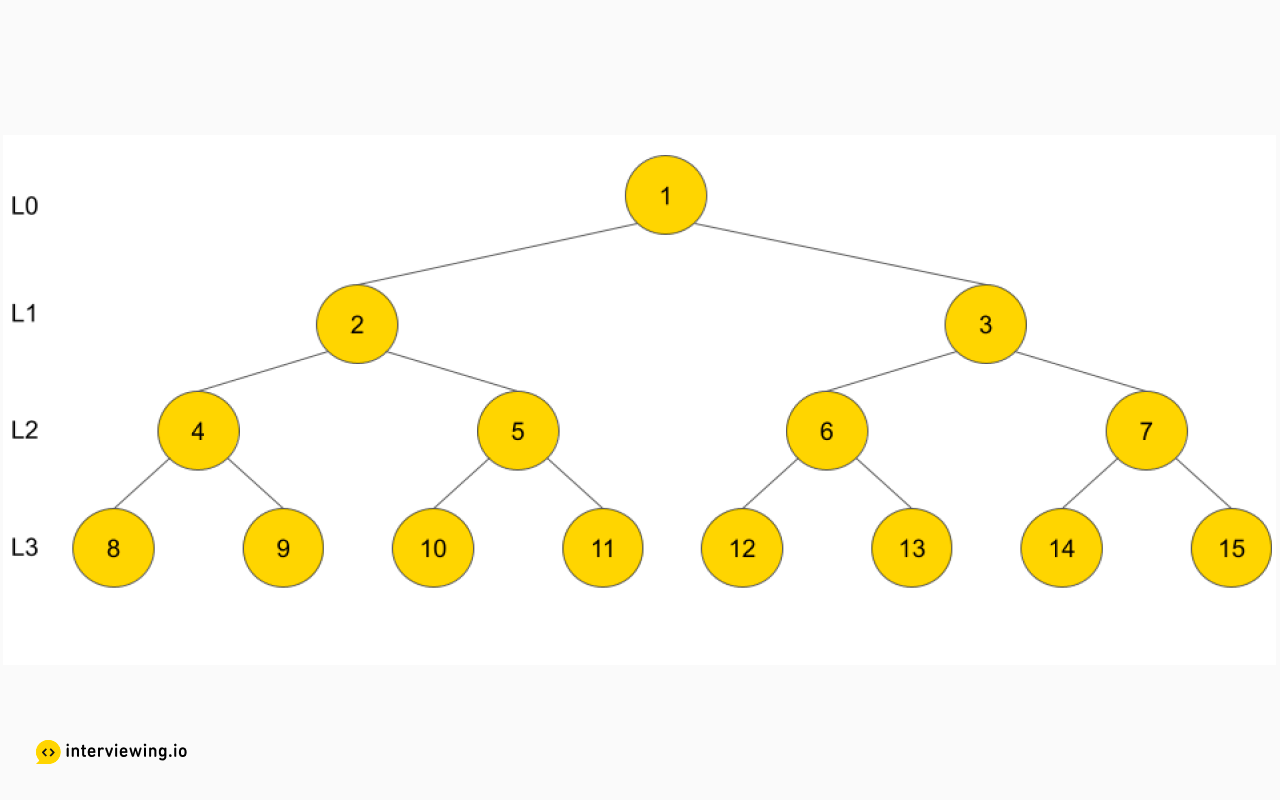Since all the levels are completely filled, we know that level 0 will have 1 node, level 1 will have 2 nodes, level 2 will have 4 nodes, and so on. So, the total number of nodes will be `2^0 + 2^1 + ... + 2^h` where `h` is the height of the tree. This equals to `2^(h+1) - 1`. Which means, in this case, we just need to find the height of the tree to calculate the number of nodes.

This is an interesting and potentially-useful realization: if the tree is completely filled (including the last level), we don't have to traverse the whole tree to know the count. We just need to know the height of the tree. Let’s keep this idea in mind while thinking of a better solution.

#### Last Level, All Nodes Are As Far Left As Possible

Let’s imagine we are playing a game. We have the complete binary tree below. We do not know which nodes in the last level are filled. Assume you have direct access to the last level’s nodes and you are allowed to reveal any of these nodes. The question is: can you identify which nodes in the last level are filled? Reveal as few nodes as you can. Remember, in a complete binary tree, all nodes are as far left as possible. Take some time to think about it.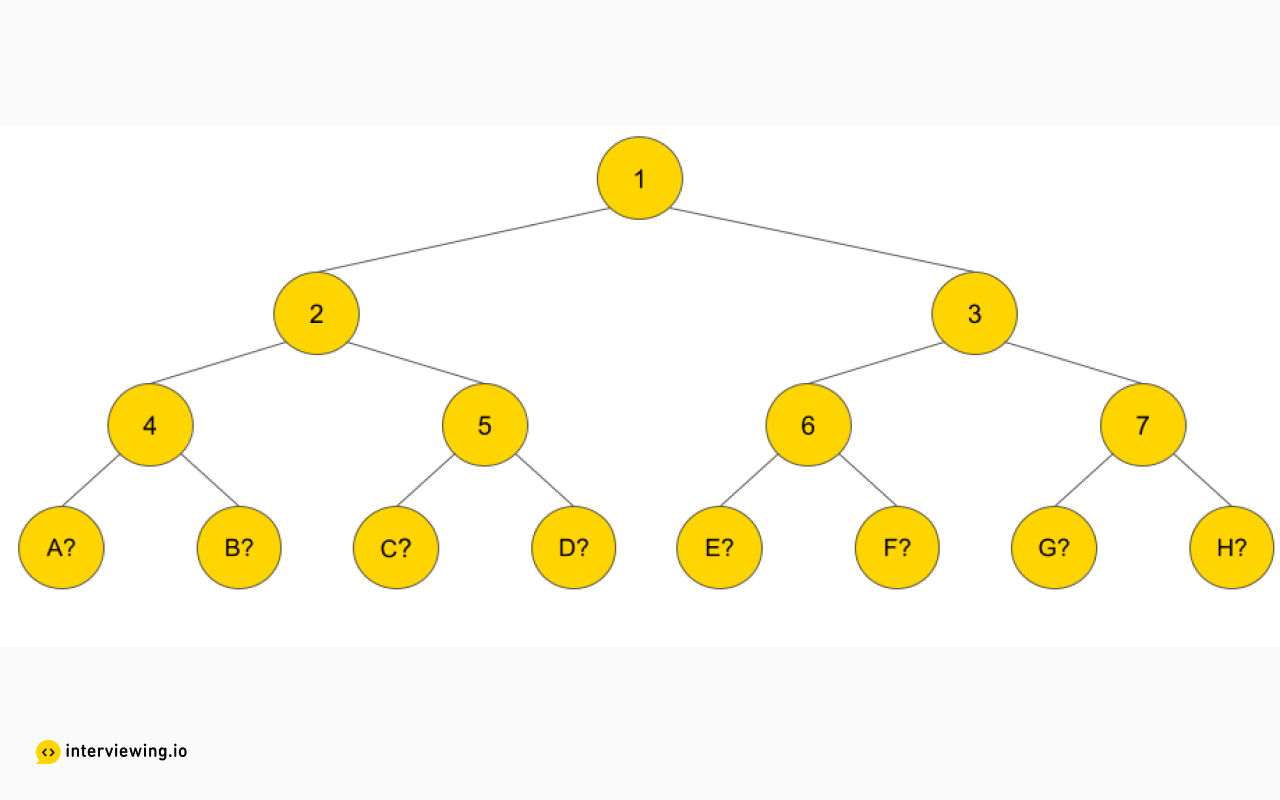One solution is to reveal node A, then B, then C until you reach an empty node. This is a linear search. Can you think of a better way?

We can start by revealing the node in the middle, if it is empty, we therefore know that everything to its right is also empty. From there we continue the search on the left side.

Does this algorithm look familiar? It should – it is binary search! By using this method we are able to make our search faster, as the time complexity would be logarithmic (`log(n)`) instead of linear (`n`).

We have a new idea now, binary search; let’s see if we can use it to improve our solution.

#### Binary Search and Complete Binary Tree

Now, if we are at the root of the tree, and we do not have direct access to the last level, how can we leverage the idea of binary search to find the rightmost node in the last level?

Let’s visualize that. We are at the root, node 1, our goal is to find the rightmost node in the last level. How can we decide if we should go left or right?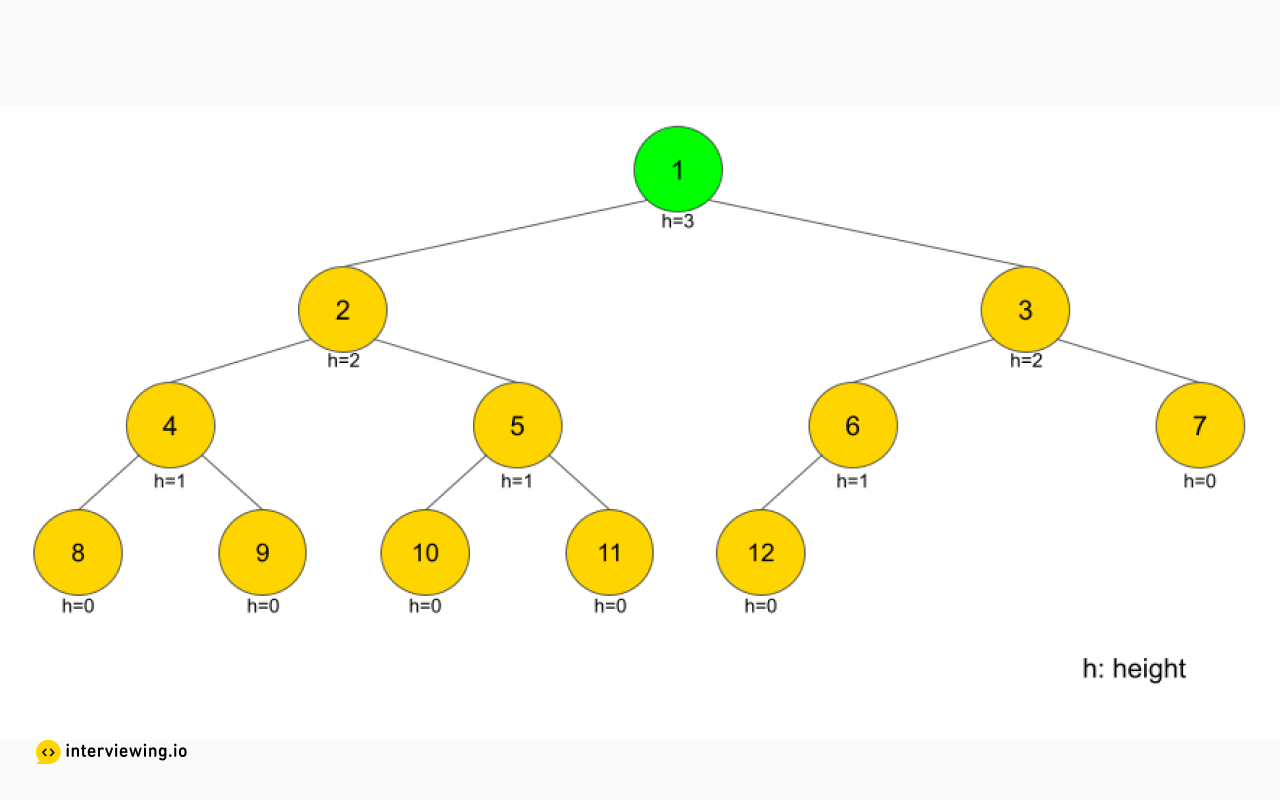Well, we can check the height of the right node, and if it is equal to the height of the current node - 1, this means that there is a path in the right tree where it will take us to a node in the last level. In such a case, we can move to the right and ignore the left tree.

Specific to our example, the current node, node 1, has height = 3 and the right node, node 3, has height = 2. This means we can go right.We now repeat the same calculation. We are at node 3 and the height of the current node is 2. The height of the right node is 0, not 1, meaning that there is no path to the last level if we go right. So we will ignore the right tree this time and move to the left.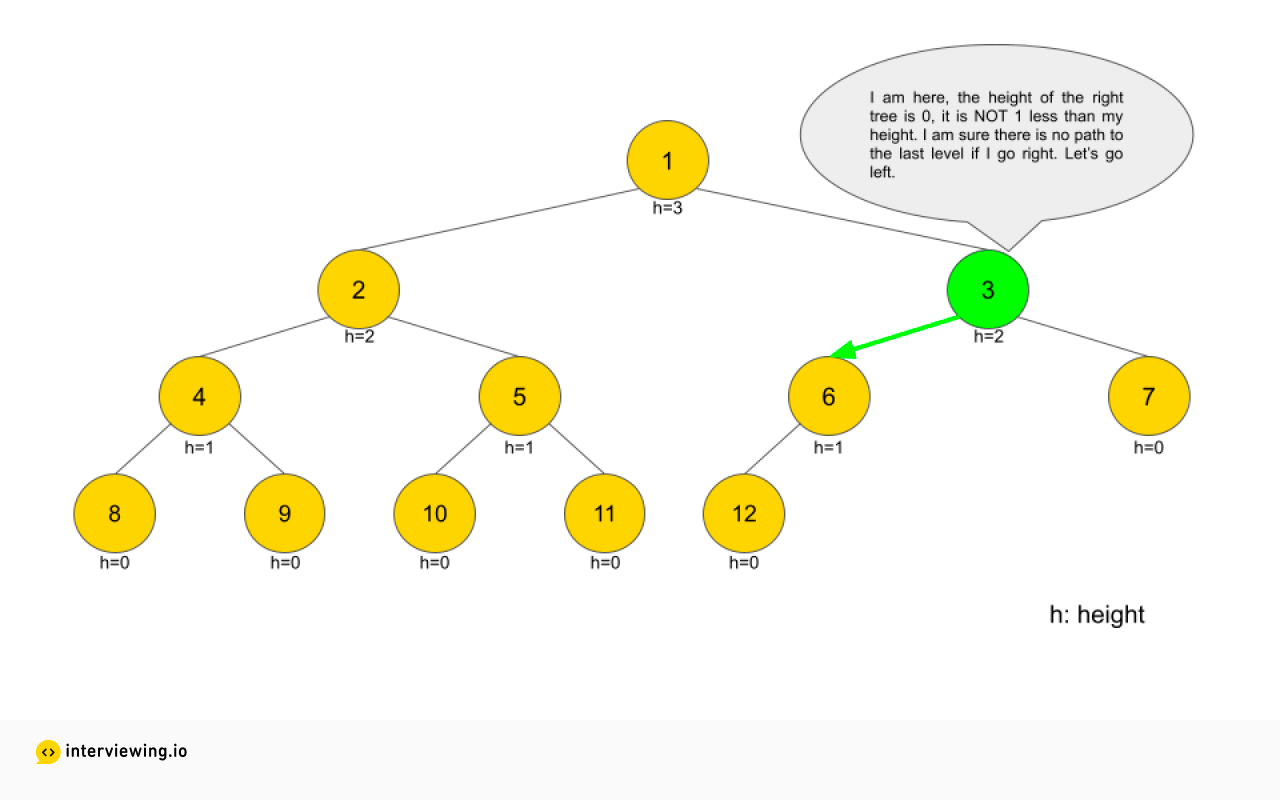And so on. We will repeat until we reach node 12.

So now that we have a basic algorithm we need to translate that logic to code. For now we will comment out the code related to counting the nodes and focus only on traversing the tree.

``````# TreeNode definition
# class TreeNode:
#    def __init__(self, data):
#        self.data = data
#        self.left = None
#        self.right = None

def calculate_height(node: TreeNode):
if not node:
return -1
height = 0
while node.left:
node = node.left
height += 1
return height

def count_nodes(root: TreeNode):
nodes_count = 0
height = calculate_height(root)

while root:
if calculate_height(root.right) == height - 1:
# left count statement
# nodes_count += ???
root = root.right
else:
# right count statement
# nodes_count += ???
root = root.left
height -= 1
return nodes_count``````

We have left two commented statements to count the nodes. When we move right, we should count all the nodes to the left. Similarly, when we move left, we should count all the nodes to the right. Can you think of how to do that? Remember what we found here.

When we go right, we know that the tree to the left is completely full, and the height of the left tree is 1 less than the height of the current node.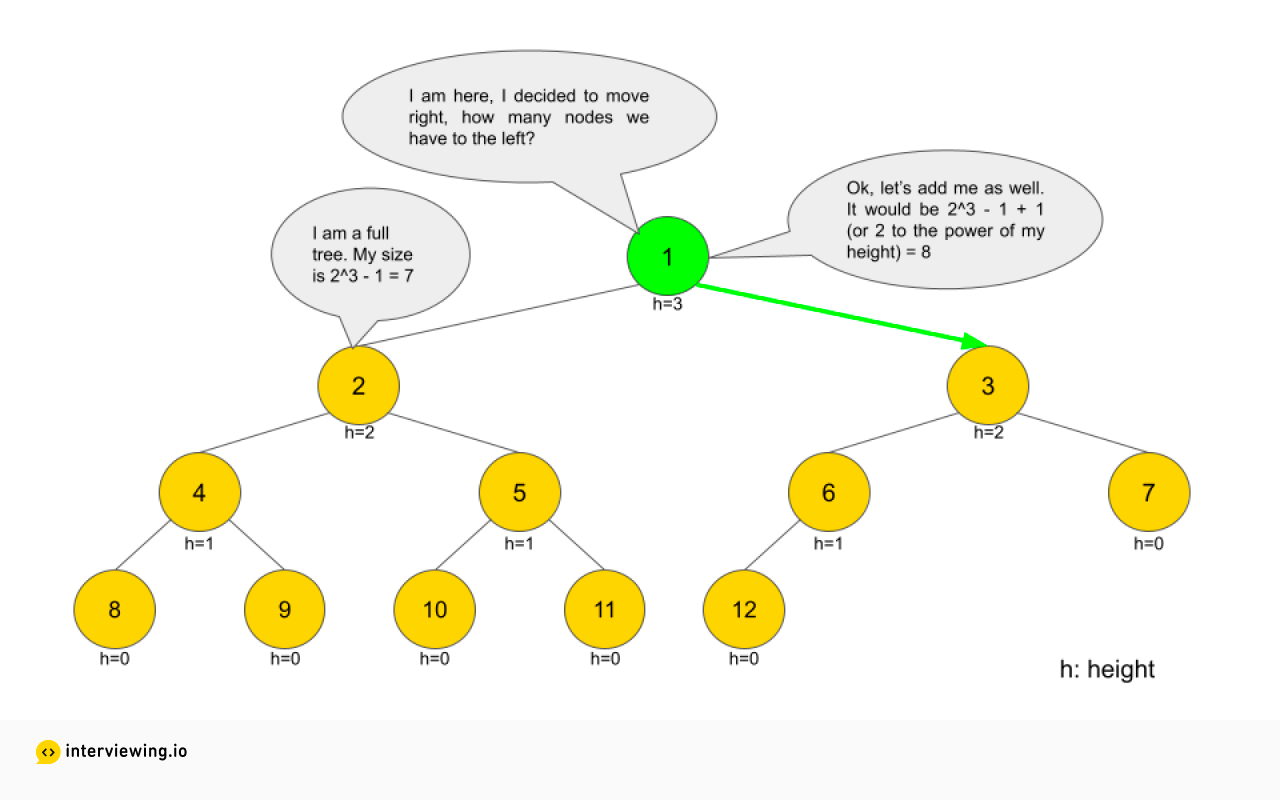This means the left count statement will be substituted with the following equation:

``````nodes_count += pow(2, height)
``````

Using the same logic, when we decide to go left, we know that the tree to the right is full. The height of the right tree will be 2 less than the height of the current node.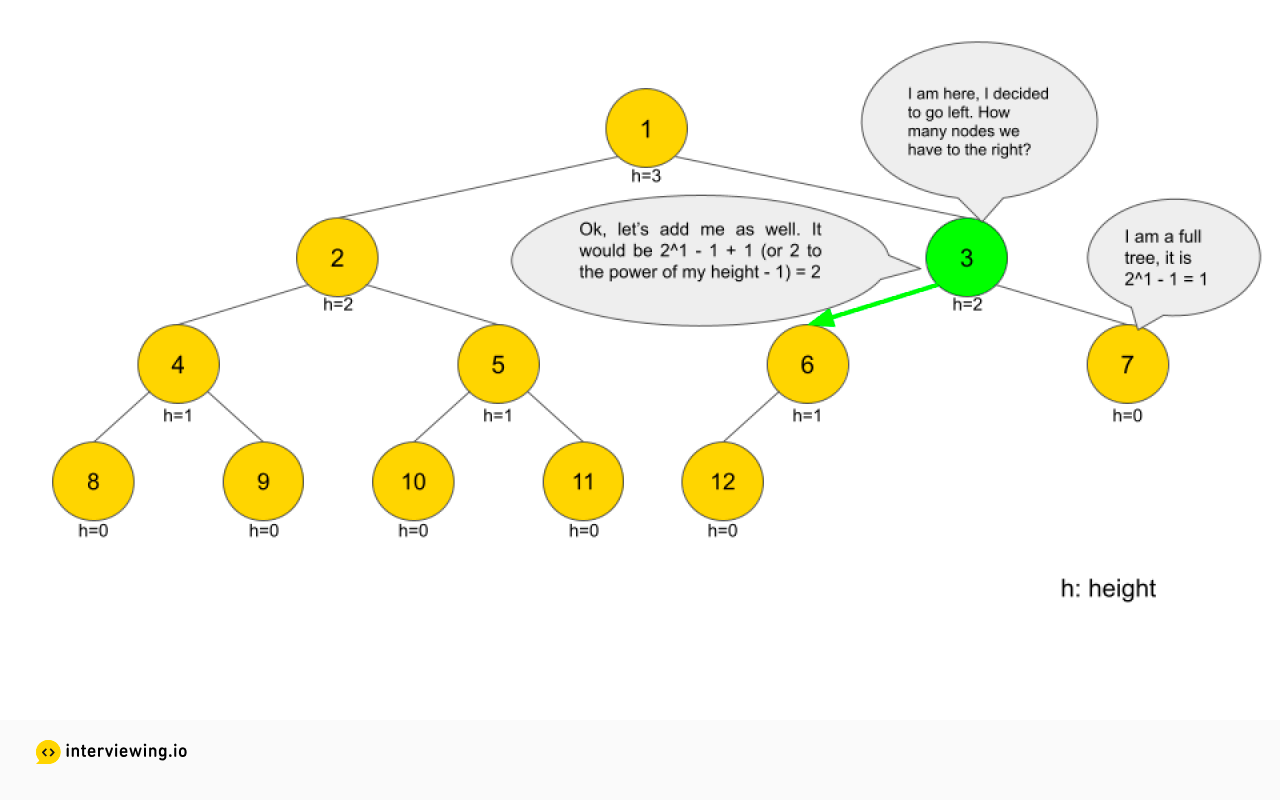This means the right count statement will be substituted with the following equation:

``````nodes_count += pow(2, height - 1)
``````

Let's add this to the code to arrive at a working implementation for Count Complete Tree Nodes.

``````# TreeNode definition
# class TreeNode:
#    def __init__(self, data):
#        self.data = data
#        self.left = None
#        self.right = None

def calculate_height(node: TreeNode):
if not node:
return -1
height = 0
while node.left:
node = node.left
height += 1
return height

def count_nodes(root: TreeNode):
nodes_count = 0
height = calculate_height(root)

while root:
if calculate_height(root.right) == height - 1:
nodes_count += pow(2, height)
root = root.right
else:
nodes_count += pow(2, height - 1)
root = root.left
height -= 1
return nodes_count
``````

#### Time/Space Complexity

• Time complexity: The `while` loop is moving from the root to the last level, Which is `h` movements, where `h` is the height of the tree. However, for each iteration of the while loop, we need to calculate the height of the right tree. Remember from the brute-force solution that `O(h)` is equivalent to `O(log2n)`, which makes the time complexity `O(h^2)` or `O((log2n)2)`, where `n` is the number of nodes.

• Space complexity: We are not using any space for our calculation so space complexity is `O(1)`.

## Practice the Count Complete Tree Nodes Problem With Our AI Interviewer

Start AI Interview